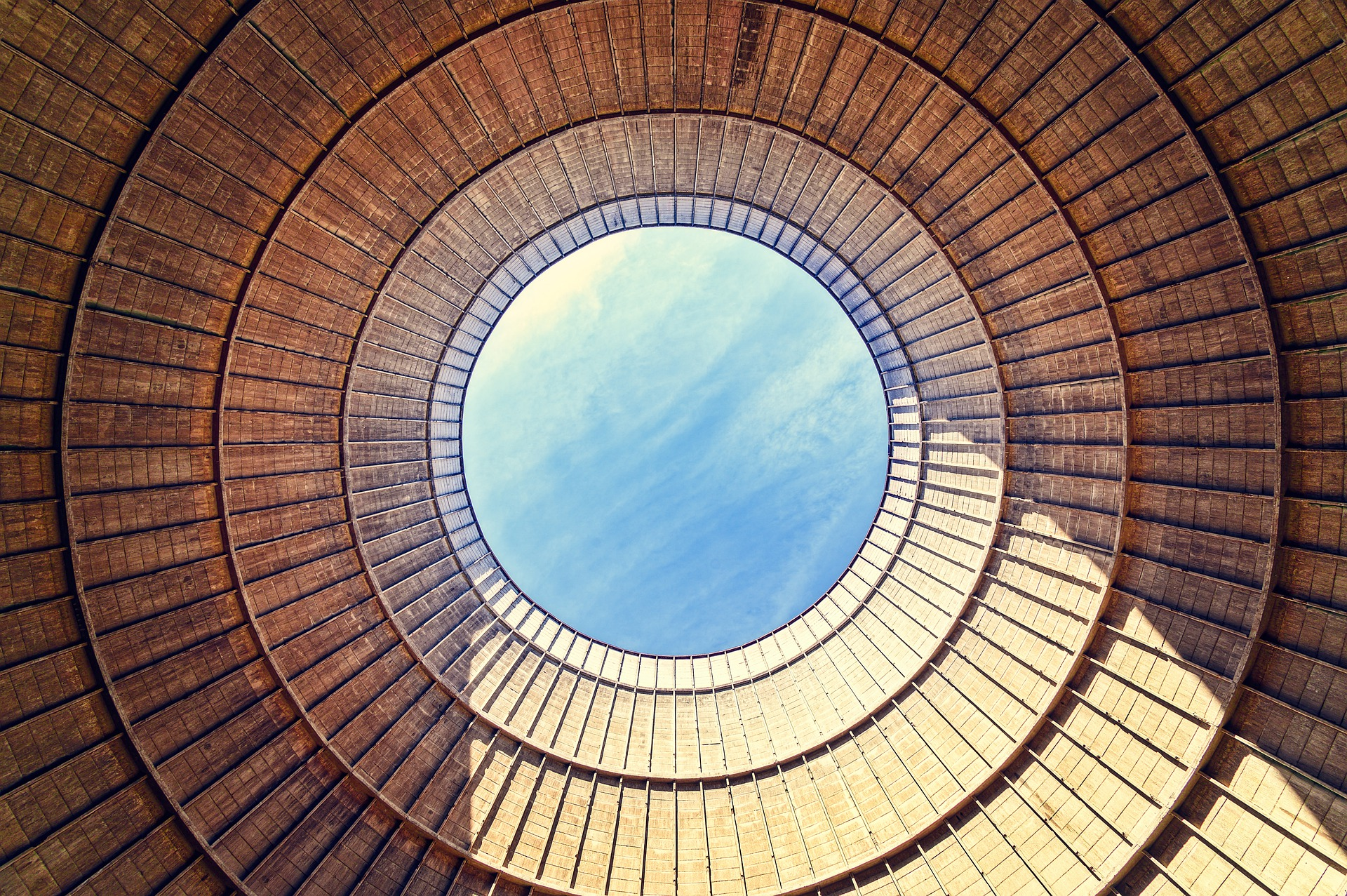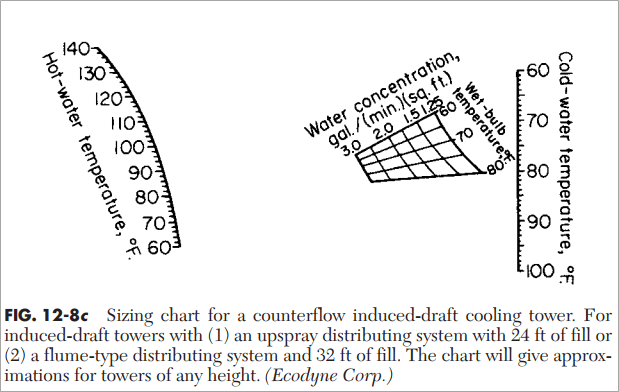## How To Estimate Insulation Thickness

In this post I want to share how to estimate insulation thickness. Charts in this post show how to estimate insulation thickness for stagnant and turbulent air conditions. Be noted, that the charts are based on neglecting the resistances caused by both the metal and the inside film at the inner wall.

Estimating Insulation Thickness for Flat Surface Read More## Sizing and Horse Power Estimation of Counterflow Induced-Draft Cooling Tower

In this post I want to share to you how to do preliminary sizing and horse power estimation for counterflow induced-draft cooling tower. Calculation in this post parallel or cross-flow cooling tower. Also they do not apply when the approach to cold water temperature is less than 5oF (2.8oC).

Approach temperature is the temperature of the water leaving the cooling tower minus ambient wet bulb temperature.

[Read more: Assessment of Cooling Tower Performance]

To do this, we need this data:

• Hot water temperature
• Cold water temperature
• Wet-bulb temperature
• Water rate

Let’s start sizing the estimation. Let say we have cooling tower with the following process data:

• Hot water temperature = 102oF
• Cold water temperature = 78oF
• Wet-bulb temperature = 70oF
• Water rate = 1000 gallon per minute

Step 1: Find water concentration by using chart

Chart below is used to find water concentration, which is expressed in gallon per minute per square feet (gal/(min.ft2)).When hot water temperature is 102oF and cold water temperature is 78oF, we get water concentration at around 2.8 gal/(min.ft2).

Step 2: Calculate required area of cooling tower

Required area of cooling tower is quantity of water circulated divided by water concentration = 1000/2.8 = 357.14 ft2.

Step 3: Find horsepower per area of cooling tower

Horsepower per area of cooling tower is estimated by using the following chart. Connecting the point representing 100 percent of standard tower performance with the turning point and extending this straight line to the horsepower scale show that it will give around 0.04 hp/ft2 of actual effective tower area. For a tower 357.14 ft2 (see Step 2), 14.6 hp is required to perform the necessary cooling.Step 4: Check if commercial tower size is less than required area

Suppose the actual commercial tower size has an area of only 300 ft2. Within reasonable limit, the shortage of actual area can be compensated by increasing air velocity through the tower. This requires boosting fan horsepower to achieve 110 percent of standard tower performance. From chart in Step 3, we found the fan horse power is 0.057 hp/ft2 of actual area, or 0.057 x 300 = 17.1 hp.

Step 5: Check if commercial tower size is more than required area

On the other hand, if the actual commercial tower size is 370 ft2, the cooling equivalent to 357.14 ft2 of standard tower area can be accomplished with less air and less fan horsepower. From figure in Step 3, the fan horsepower for a tower operating at 90 percent of standard performance is 0.028 hp/ft2 of actual tower area, or 370 x 0.028 = 10.4 hp.

Reference:

Perry’s Chemical Engineers’ Handbook.## Hydrate Prediction using Vapor-Solid Equilibrium Constant

In previous post, hydrate in natural gas system can be estimated by two approaches, which are approximate method and analytical method. Approximate method used when gas composition is unknown. It uses pressure-temperature correlation to predict hydrate formation temperature.

In this post, I want to share how to predict hydrate formation using analytical method, which uses vapor-solid equilibrium constant. Vapor-solid equilibrium constant is expressed as below: